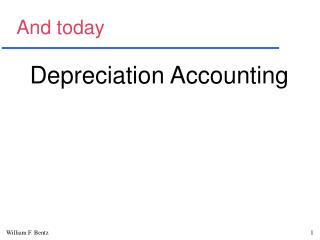# And today - PowerPoint PPT PresentationDownload PresentationAnd today

And today
Download Presentation## And today

- - - - - - - - - - - - - - - - - - - - - - - - - - - E N D - - - - - - - - - - - - - - - - - - - - - - - - - - -
##### Presentation Transcript

1. And today Depreciation Accounting

2. Depreciation--definition Depreciation is the process by which the cost of property, plant & equipment is either matched with revenues, or allocated to the periods of benefit. As an allocation process, the primary emphasis is on income measurement and reporting, and secondarily on the valuation of depreciable assets for SFP purposes.

3. Principles • The total depreciation to be taken is equal to the cost of an asset less its value at the end of its useful life. • The method used to depreciate an asset should reflect the stream of the benefits derived by using the asset and the resulting net book values over the life of the asset.

4. Principles • At any point in the life of a depreciable asset, the net book value of the asset should be less than or equal to the undiscounted cash flows that can be derived from that asset through its use or sale. This principle constrains the use of the depreciation method used.

5. Depreciation considerations • The allocation process must be systematic and rational. I interpret rational to mean we should communicate clearly to investors any changes in expected profitability.

6. ★★★★ 4 criteria to consider • Relationship of the usage of an asset to the decline in its remaining economic life • Impact on profitability measures • Total amount of depreciation taken • Resulting valuation of the asset

7. Models in preference order • Where there is a cause-effect relationship between a measure of work done and the resulting decline in the economic life of a depreciable asset, then that relationship should be used to compute depreciation expense. [i.e., use the production method]

8. Cause-effect examples • Operation of a jet engine which by regulation must be overhauled after a certain number of hours of use. • Cutting and drilling tools replaced after a specified amount of use to avoid problems caused by wear. • Any equipment that wears out with usage.

9. Bottom-line... • When a measurable cause-effect relationship exists, use it to depreciate the associated assets.

10. Profitability impact (2nd. best) • Equalize (average) the reported gross margin generated by the asset over the useful life of the asset (formula methods) • Equalize the accounting rate of return on net book value over the life of the asset (interest--aka. annuity--method)

11. Implications of the above • The most appropriate method is context-specific • There is no “best” method of depreciation • From a financial reporting perspective, the total depreciation is what counts. • In each situation, several methods may be equally appropriate

12. Practice issues • The most difficult issue is predicting useful lives • Many assets cannot be related directly to any revenue-generating activities • Some companies are more aggressive than others in reporting income.

13. Notation we will use • Let C = the portion of the cost of a depreciable asset that may be capitalized, • Di = the depreciation expense for year i, • S = the residual or net scrap value of the depreciable asset, • n = the planned economic life of the depreciable asset, and • Cfi= net cash flow in period i

14. Production method • Formula • With the production method, depreciation expense varies with the amount of production.

15. Straight-line method • Formula: • If C, S, and n do not change, the annual depreciation is constant over the life of an asset.

16. Sum-of-the-years’ digits (SYD)  Formula:  For the SYD method, the rate declines linearly and the base remains constant, so the depreciation expense declines linearly.

17. Declining balance (DB) method Formula: In the DB method, the depreciation rate is unchanged, but the base declines exponentially. The resulting depreciation expense declines exponentially.

18. DB method rates • There are three rates, two arbitrary and one calculated rate that can be used with the DB method: •  2 times the st.-line rate r = 2(1/n) •  1.5 times the st.-line rate r = 1.5(1/n) •  a calculated rate r, where

19. St.-line/DB hybrid method • In addition to the above methods, the St.-line/declining balance hybrid balance method involves the use of one of the arbitrary-rate DB methods and a switch to straight-line during the first year the depreciation is greater with the straight-line method.

20. The interest method • Formula • Interest method depreciation is a function of the IRR “r” and the cash flows. Depreciation can even be negative for one or more periods.

21. THE END